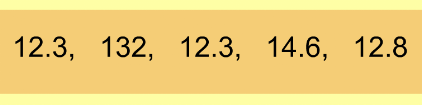Finding the Outlier 1
Set 1 (10 Questions)
Students organize, represent, and interpret numerical and categorical data and clearly communicate their findings. Identify the mode(s) for sets of categorical data and any apparent outliers for numerical data sets.
From Mr. Anker Tests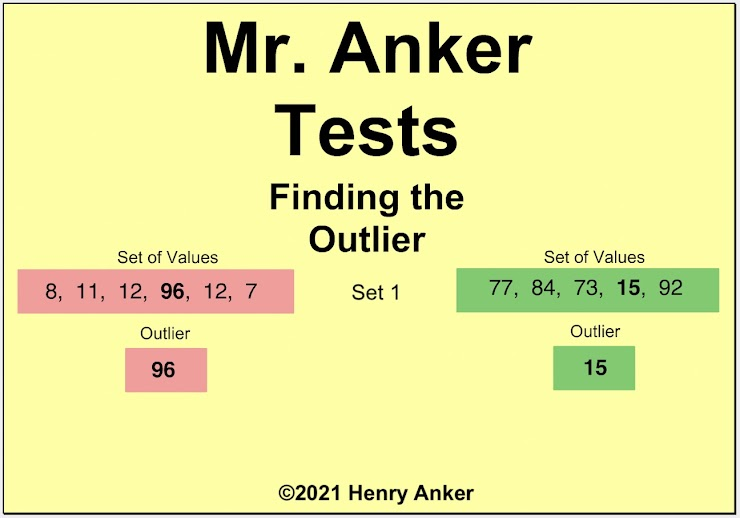Finding the Outlier Help Slide 1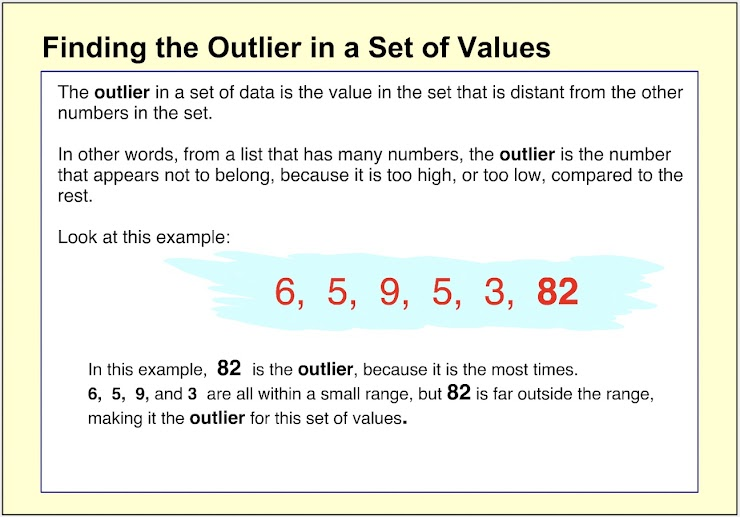Finding the Outlier Help Slide 2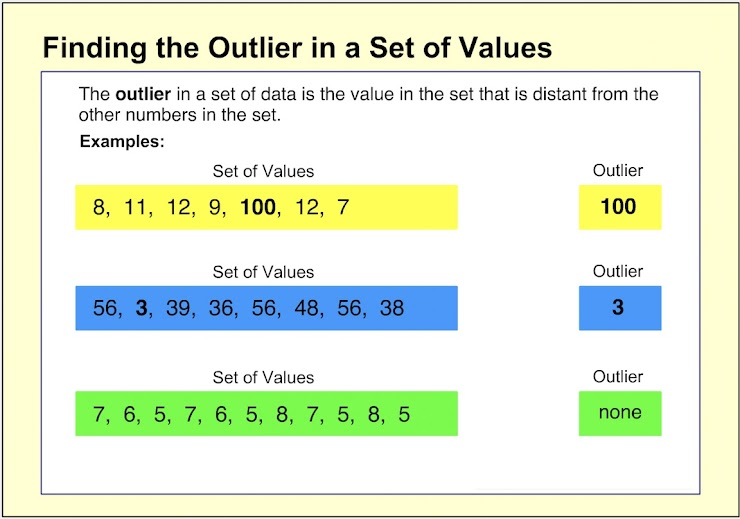Finding the Outlier Help Slide 3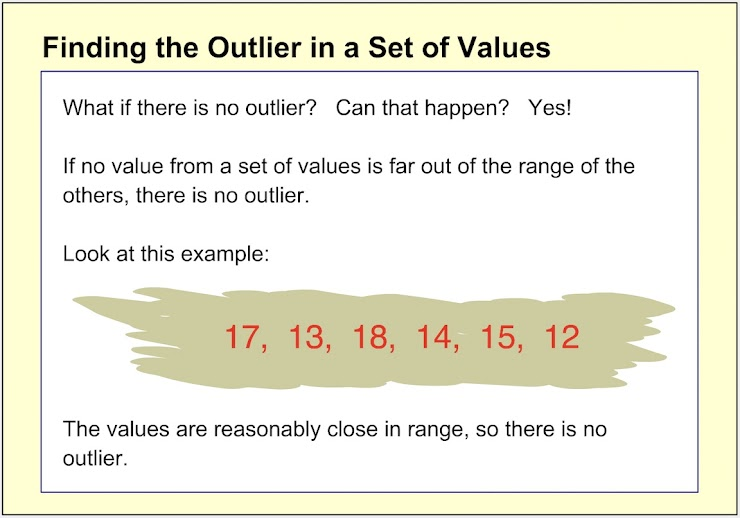Finding the Outlier Help Slide 4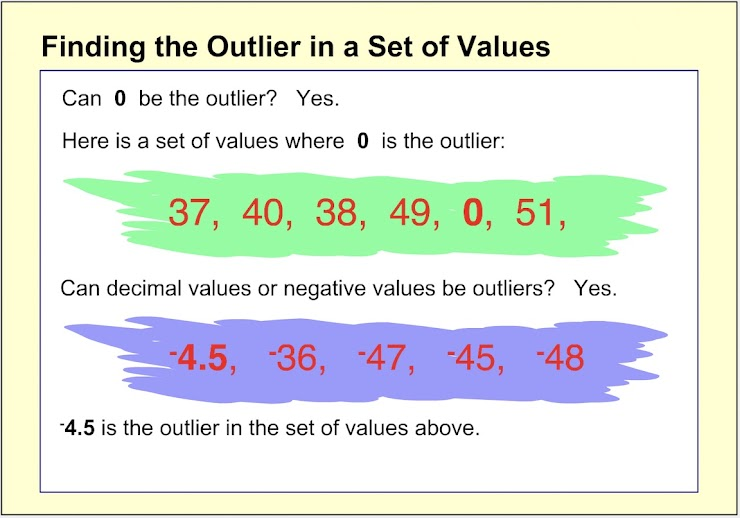1. Which choice below is the outlier for the set of values shown? *
1 point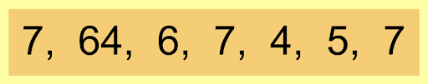Remember that the outlier value, if there is one, is a value that is far, far higher or lower than the rest of the numbers in a set.
2. Which choice below is the outlier for the set of values shown? *
1 point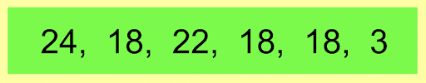3. Which choice below is the outlier for the set of values shown? *
1 point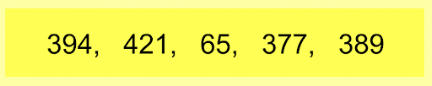4. Which choice below is the outlier for the set of values shown? *
1 point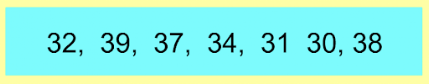5. On the line below, enter the outlier for the set of values shown. *
1 point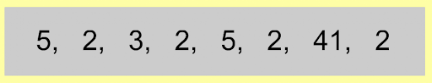6. On the line below, enter the outlier for the set of values shown. *
1 point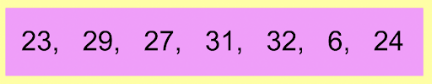7. On the line below, enter the outlier for the set of values shown. *
1 point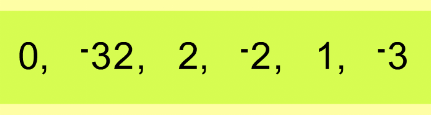8. On the line below, enter the outlier for the set of values shown. *
1 point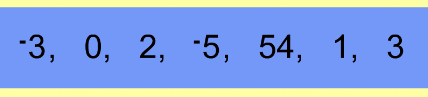9. On the line below, enter the outlier for the set of values shown. *
1 point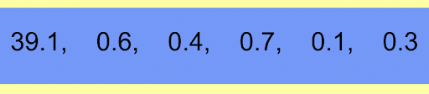10. On the line below, enter the outlier for the set of values shown. *
1 point